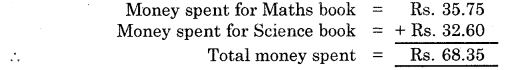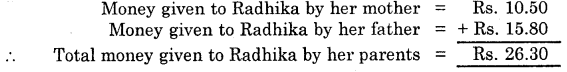# HBSE 6th Class Maths Solutions Chapter 8 Decimals Ex 8.5

Haryana State Board HBSE 6th Class Maths Solutions Chapter 8 Decimals Ex 8.5 Textbook Exercise Questions and Answers.

## Haryana Board 6th Class Maths Solutions Chapter 8 Decimals Exercise 8.5

Question 1.
Find the sum in each of the following :
(a) 0.007 + 8.5 + 30.08
(b) 15 + 0.632 + 13.8
(c) 27.076 + 0.55 + 0.004
(d) 25.65 + 9.005 + 3.7
(e) 0.75 + 10.425 + 2
(f) 280.69 + 25.2 + 38.
Solution:Question 2.
Rashid spent Rs. 35.75 for Maths book and Rs. 32.60 for Science book. Find the total amount spent by Rashid.
Solution:Question 3.
Radhika’s mother gave her Rs. 10.50 and her father gave her Rs. 15.80, find the total amount given to Radhika by her parents.
Solution:Question 4.
Narseen bought 3 m 20 cm cloth for her shirt and 2 m 5 cm cloth for her trousers. Find the total length of cloth bought by her.
Solution:
Length of cloth bought by Narseen for her shirt = 3 m 20 cm = 3.20 m
Length of cloth bought by her for trousers = 2 m 5 cm = 2.05 m ,
∴  Total length of cloth bought by her 3.20 m + 2.05 m
= 5.25 m = 5 m 25 cm.Question 5.
Wilson bought 2 m 50 cm cloth for his kurta and 1 m 25 cm cloth for his pyjama. Find the total length of cloth bought by him.
Solution:
Length of cloth bought by Wilson for his kurta = 2 m 50 cm = 2.50 m
Length of cloth bought by him for his pyjama = 1 m 25 cm = 1.25 m
∴ Total length of cloth bought by him = 2.50 m + 1.25 m